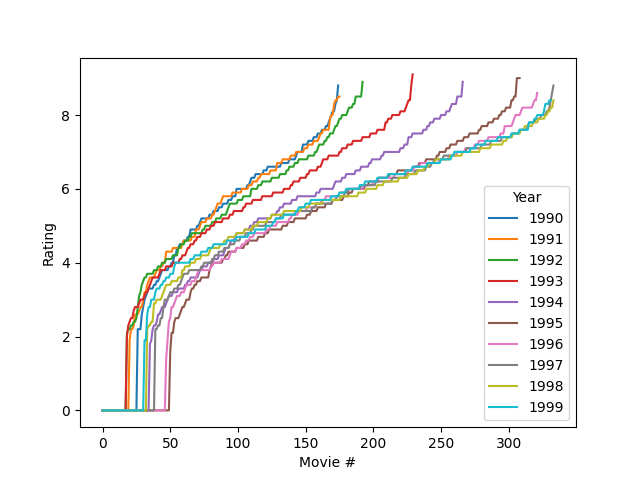# Statistics: Exercises and Solutions

## A three-way Repeated Measures ANOVA

### Exercise

Above you have seen how to conduct a two-way repeated measures ANOVA with this dataset from Zhou et al. (2020). But the data contains a third factor: congruency. First, run a three-way repeated measures ANOVA with target-match, distractor-match, and congruency as independent variables, and search accuracy as dependent variable. Next, plot the results in a two-panel plot, where the left subplot shows the effect of distractor and target match for congruent trials, while the right subplot shows this for incongruent trials.

### Solution

``````from pandas import pivot_table
from datamatrix import io
from datamatrix import operations as ops
from statsmodels.stats.anova import AnovaRM
import seaborn as sns

# Read the data and pass it to AnovaRM
aov = AnovaRM(
dm,
depvar='search_correct',
subject='subject_nr',
within=[
'target_match',
'distractor_match',
'congruency'
],
aggregate_func='mean'
).fit()
print(aov)
# Now plot the data!
dm_congruent, dm_incongruent = ops.split(dm.congruency, 1, 0)
plt.subplot(1, 2, 1)
plt.title('Congruent')
sns.pointplot(
x='target_match',
y='search_correct',
hue='distractor_match',
data=dm_congruent
)
plt.ylim(0.75, 1)
plt.xlabel('Target match')
plt.ylabel('Search accuracy (proportion)')
plt.legend(title='Distractor match')
plt.subplot(1, 2, 2)
plt.title('Incongruent')
sns.pointplot(
x='target_match',
y='search_correct',
hue='distractor_match',
data=dm_incongruent
)
plt.ylim(0.75, 1)
plt.xlabel('Target match')
plt.ylabel('Search accuracy (proportion)')
plt.legend(title='Distractor match')
plt.show()
``````

Output:

``````                                Anova
======================================================================
F Value Num DF  Den DF Pr > F
----------------------------------------------------------------------
target_match                              6.7339 1.0000 34.0000 0.0139
distractor_match                         13.9729 1.0000 34.0000 0.0007
congruency                               15.1063 1.0000 34.0000 0.0004
target_match:distractor_match             7.1687 1.0000 34.0000 0.0113
target_match:congruency                  16.2760 1.0000 34.0000 0.0003
distractor_match:congruency               2.9842 1.0000 34.0000 0.0932
target_match:distractor_match:congruency  1.2566 1.0000 34.0000 0.2702
======================================================================
``````You're done with this section!